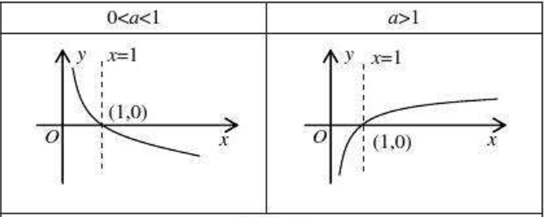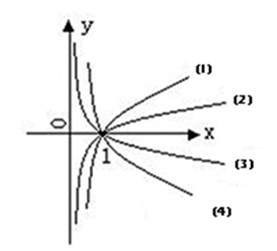• ## python绘制对数函数

千次阅读 2020-02-18 11:16:47
如何利用python的matplotlib模块绘制对数函数


python绘制对数函数
a>1完整代码效果图
a>1完整代码效果图
对数函数vs指数函数完整代码效果图
参考文献

上一期，我们在探讨对数函数性质的时候，我们画了几个非常漂亮的图，这一期，就把画图的python放上来，设对数函数为

y

=

l

o

g

a

x

y=log_a x

其中a>0，且a≠1。对应的指数函数为

y

=

a

x

y=a^x

。现在来分情况画出对数函数的图像
a>1
完整代码
# -*- coding: utf-8 -*-
"""
Created on Mon Feb 17 17:32:49 2020
project name:draw_logarithm_figure
@author: 帅帅de三叔
"""
import math
import numpy as np
import matplotlib.pyplot as plt
import mpl_toolkits.axisartist as axisartist #导入坐标轴加工模块
plt.rcParams['font.sans-serif']=['SimHei']
plt.rcParams['axes.unicode_minus']=False

fig=plt.figure(figsize=(6,4)) #新建画布
ax=axisartist.Subplot(fig,111) #使用axisartist.Subplot方法创建一个绘图区对象ax

def logarithm_func(x, a=1/2): #定义指数函数
y=math.log(x, a)
return y

X=np.linspace(0.01, 4, 100) #构造自变量组
Y=[logarithm_func(x) for x in X] #求函数值
ax.plot(X, Y, label=r'$0<a<1$') #绘制指数函数
ax.scatter(1, 0, color='red')
plt.legend()
plt.show()

print(max(X), max(Y)) #测试一下自变量最大值和因变量最大值，为后面的坐标轴设置依据
ax.axis[:].set_visible(False) #隐藏原来的实线矩形
ax.axis["x"]=ax.new_floating_axis(0, 0, axis_direction="bottom") #添加x轴
ax.axis["y"]=ax.new_floating_axis(1, 0, axis_direction="bottom") #添加y轴

ax.axis["x"].set_axisline_style("-|>", size=1.0) #给x坐标轴加箭头
ax.axis["y"].set_axisline_style("-|>", size=1.0) #给y坐标轴加箭头

ax.annotate(s='x', xy=(max(X), 0), xytext=(max(X)+1, 0.3)) #标注x轴
ax.annotate(s='y', xy=(0, 1.0), xytext=(-0.2, max(Y)+1)) #标注y轴

plt.xlim(-1, 5) #设置横坐标范围
plt.ylim(-5, 3) #设置纵坐标范围
X_lim=np.arange(int(min(X)), max(X)+1, 1)
ax.set_xticks(X_lim) #设置x轴刻度
Y_lim=np.arange(-3, max(Y)+1, 1)
ax.set_yticks(Y_lim) #设置y轴刻度
ax.annotate(s=r'$y=log_a x$',xy=(1, 1), xytext=(1, 1)) #r'$y=(\frac{1}{2})^x$'

效果图a>1
完整代码
# -*- coding: utf-8 -*-
"""
Created on Mon Feb 17 17:32:49 2020
project name:draw_logarithm_figure
@author: 帅帅de三叔
"""
import math
import numpy as np
import matplotlib.pyplot as plt
import mpl_toolkits.axisartist as axisartist #导入坐标轴加工模块
plt.rcParams['font.sans-serif']=['SimHei']
plt.rcParams['axes.unicode_minus']=False

fig=plt.figure(figsize=(6,4)) #新建画布
ax=axisartist.Subplot(fig,111) #使用axisartist.Subplot方法创建一个绘图区对象ax

def logarithm_func(x, a=2): #定义指数函数
y=math.log(x, a)
return y

X=np.linspace(0.01, 4, 100) #构造自变量组
Y=[logarithm_func(x) for x in X] #求函数值
ax.plot(X, Y, label=r'$a>1$') #绘制指数函数
ax.scatter(1, 0, color='red')
plt.legend()
plt.show()

print(max(X), max(Y)) #测试一下自变量最大值和因变量最大值，为后面的坐标轴设置依据
ax.axis[:].set_visible(False) #隐藏原来的实线矩形
ax.axis["x"]=ax.new_floating_axis(0, 0, axis_direction="bottom") #添加x轴
ax.axis["y"]=ax.new_floating_axis(1, 0, axis_direction="bottom") #添加y轴

ax.axis["x"].set_axisline_style("-|>", size=1.0) #给x坐标轴加箭头
ax.axis["y"].set_axisline_style("-|>", size=1.0) #给y坐标轴加箭头

ax.annotate(s='x', xy=(max(X), 0), xytext=(max(X)+1, 0.3)) #标注x轴
ax.annotate(s='y', xy=(0, 1.0), xytext=(-0.2, max(Y)+1)) #标注y轴

plt.xlim(-1, 5) #设置横坐标范围
plt.ylim(-5, 3) #设置纵坐标范围
X_lim=np.arange(int(min(X)), max(X)+1, 1)
ax.set_xticks(X_lim) #设置x轴刻度
Y_lim=np.arange(-3, max(Y)+1, 1)
ax.set_yticks(Y_lim) #设置y轴刻度
ax.annotate(s=r'$y=log_a x$',xy=(1, 1), xytext=(1, 1)) #r'$y=(\frac{1}{2})^x$'

效果图两个代码对比，可以看到，仅改了参数a的初始值和label标签，因为两个函数的定义域和值域是一致的。
对数函数vs指数函数
这两个函数虽然互为反函数，但是定义域和值域不一致，所以需要在定义函数的时候给出不同的自变量取值范围，否则会出现math domain error 。
完整代码
# -*- coding: utf-8 -*-
"""
Created on Tue Feb 18 08:49:21 2020
project name:logarithm_vs_exponential
@author: 帅帅de三叔
"""
import math
import numpy as np
import matplotlib.pyplot as plt
import mpl_toolkits.axisartist as axisartist #导入坐标轴加工模块
plt.rcParams['font.sans-serif']=['SimHei']
plt.rcParams['axes.unicode_minus']=False

fig=plt.figure(figsize=(6,4)) #新建画布
ax=axisartist.Subplot(fig,111) #使用axisartist.Subplot方法创建一个绘图区对象ax

def logarithm_func(x, a=2): #定义指数函数
y=math.log(x, a)
return y

def exponential_func(x, a=2): #定义指数函数
y=math.pow(a, x)
return y

X=np.linspace(0.01, 4, 100) #构造自变量组
Y=[logarithm_func(x) for x in X] #求函数值
ax.plot(X, Y, label='对数函数') #绘制指数函数
ax.scatter(1, 0, color='red')

X1=np.linspace(-4, 4, 100)
Y1=[exponential_func(x) for x in X1]
ax.plot(X1, Y1, label='指数函数')
ax.scatter(0, 1, color='red')

ax.plot(X1, X1, color='green', label=r'$y=x$')
plt.legend(loc=2)
plt.show()

print(max(X), max(Y)) #测试一下自变量最大值和因变量最大值，为后面的坐标轴设置依据
ax.axis[:].set_visible(False) #隐藏原来的实线矩形
ax.axis["x"]=ax.new_floating_axis(0, 0, axis_direction="bottom") #添加x轴
ax.axis["y"]=ax.new_floating_axis(1, 0, axis_direction="bottom") #添加y轴

ax.axis["x"].set_axisline_style("-|>", size=1.0) #给x坐标轴加箭头
ax.axis["y"].set_axisline_style("-|>", size=1.0) #给y坐标轴加箭头

ax.annotate(s='x', xy=(max(X), 0), xytext=(max(X)+1, 0.3)) #标注x轴
ax.annotate(s='y', xy=(0, 1.0), xytext=(-0.2, 5)) #标注y轴

plt.xlim(-5, 5) #设置横坐标范围
plt.ylim(-5, 5) #设置纵坐标范围
X_lim=np.arange(-4, 4, 1)
ax.set_xticks(X_lim) #设置x轴刻度
Y_lim=np.arange(-4, 4, 1)
ax.set_yticks(Y_lim) #设置y轴刻度

效果图只需要在定义指数函数和对数函数的时候给a赋予不同的参数便可出现不同的形状，下图是a=1/2 时的效果图如果你不会写代码或者还有什么不懂的欢迎来“三行科创”微信公众号留言，同时交流群免费向大家开放，入群讲缘分。
参考文献
1，https://blog.csdn.net/zengbowengood/article/details/104338878 2，https://blog.csdn.net/zengbowengood/article/details/102862072 3，https://blog.csdn.net/zengbowengood/article/details/104360150 4，https://blog.csdn.net/zengbowengood/article/details/104260155 5，https://matplotlib.org/api/_as_gen/matplotlib.pyplot.html 6，https://blog.csdn.net/qq_17528659/article/details/82152530展开全文python matplotlib
• 本文主要介绍CTR场景中的对数损失函数和交叉熵损失函数，以及对数损失函数取值范围，如果觉得对你有帮助，文末「分享」「点赞」「在看」来一波，点击「阅读原文」获取精彩比例PDF下载链接。 极大似然估计 在...

本文主要介绍CTR场景中的对数损失函数和交叉熵损失函数，以及对数损失函数的取值范围，如果觉得对你有帮助，文末「分享」「点赞」「在看」来一波，点击「阅读原文」获取精彩比例PDF下载链接。

极大似然估计
在统计学领域，有两种对立的思想学派：贝叶斯学派和经典学派（频率学派），他们之间最大的区别是如何看待被估计的参数。贝叶斯学派的观点是将其看成是已知分布的随机变量，而经典学派的观点是将其看成未知的待估计的常量。
极大似然估计属于经典学派的一种。通俗来说，极大似然估计就是利用已知的样本结果信息，反推最大概率出现这些结果的参数信息。
举一个通俗点的例子来说明一下什么是极大似然估计。比如我们在网上发现了两篇重复率比较高的文章，但作者ID并不是一个人，我们推测这两个作者之间存在一定的“关系”，经过对比文章的发布时间和其他文章的写作风格，我们认为存在极大的可能是A抄袭了B的文章。这个推测的过程就称为似然，得到可能结论就是极大似然估计。
极大似然估计中采样需满足一个重要的假设，就是所有的采样都是独立同分布的。在进行参数计算的过程中，首先假设样本服从某种概率分布，在利用已知的样例数据对参数进行估计。
假设

P

(

x

∣

c

)

P(x|c)

具有确定的概率分布形式，且被参数

θ

c

\theta_c

唯一确定，则任务就是利用训练集

D

D

来估计参数

θ

c

\theta_c

。

θ

c

\theta_c

对于训练集

D

D

中第

c

c

类样本组成的集合

D

c

D_c

的似然（likelihood）为：

P

(

D

c

∣

θ

c

)

=

∏

x

∈

D

c

P

(

x

∣

θ

c

)

P(D_c|\theta_c)= \prod _{x\in D_c} P(x|\theta_c)

如果使用连乘进行参数估计在计算时很不方便，所以这里取了一个对数，即对数似然估计：

L

L

(

θ

c

)

=

l

o

g

P

(

D

c

∣

θ

c

)

=

∑

x

∈

D

c

l

o

g

P

(

x

∣

θ

c

)

LL(\theta_c)= log \, P(D_c|\theta_c)= \sum _{x\in D_c} log \, P(x|\theta_c)

于是

θ

c

\theta_c

的极大似然估计为：

θ

c

^

=

a

r

g

m

a

x

θ

c

L

L

(

θ

c

)

\hat{ \theta_c } = \underset{\theta_c}{arg \, max} \, LL(\theta_c)

参数估计结果的准确性严重依赖于所假设的概率分布形式是否符合潜在的真实分布。

对数损失函数和交叉熵损失函数
对数损失函数（Log loss function）和交叉熵损失函数（Cross-entroy loss funtion）在很多文献内是一致的，因为他们的表示式的本质是一样的。
log loss function表达式：

l

o

g

l

o

s

s

=

−

1

n

∑

i

=

1

n

(

y

i

l

o

g

(

p

i

)

+

(

1

−

y

i

)

l

o

g

(

1

−

p

i

)

)

logloss = - \frac{1}{n}\sum_{i=1}^{n} (y_i log (p_i) + (1-y_i) log(1-p_i))

其中

y

i

y_i

是第

i

i

个样本的真实标签，

p

i

p_i

是第

i

i

个样本预测为正样本的概率。
cross-entropy loss function表达式：

c

r

o

s

s

e

n

t

r

o

p

y

l

o

s

s

=

−

1

m

∑

i

=

1

m

∑

j

=

1

n

y

i

j

l

o

g

(

p

(

x

i

j

)

)

crossentropy\,loss = -\frac{1}{m} \sum_{i=1}^{m} \sum_{j=1}^{n} y_{ij} \, log (p(x_{ij}))

其中

m

m

表示样本数，

n

n

表示样本所属的不同类别个数，

y

i

j

y_{ij}

表示样本

i

i

所属类别

j

j

，

p

(

x

i

j

)

p(x_{ij})

表示预测的样本

i

i

属于类别

j

j

的概率。
从上述的表达式中看，两者的损失函数本质是一样的，但是这里需要注意的是通常情况下，这两种损失函数所对应的上一层结构不同，log loss经常对应的是Sigmoid函数的输出，用于二分类问题；而cross-entropy loss经常对应的是Softmax函数的输出，用于多分类问题。
所以在神经网络中精彩使用cross-entropy作为评判参数优化的函数，而在二分类的场景下精彩使用log loss作为评判参数优化的函数。
但是其实log loss也可以应用在多分类，这时候就和cross-entropy loss应用在多分类没有什么差别了。

log loss function 和 cross-entropy loss function本质上没有什么区别，无论是在二分类还是多分类场景下都可以进行应用，只不过大多数人的应用习惯不同而已。

对数损失优化取值范围
上一小节中的log loss表达式可以转换为：

l

o

g

l

o

s

s

=

−

∑

i

=

1

n

(

y

i

n

l

o

g

(

p

i

)

+

1

−

y

i

n

l

o

g

(

1

−

p

i

)

)

logloss = - \sum_{i=1}^{n} (\frac{y_i}{n} log (p_i) + \frac{1-y_i}{n} log(1-p_i))

假如我们现在有个训练集，100万个数据点，其中10万个为阳性，那么总体上每个样本为1的概率可近似认为是0.1。通常来说，测试集的分布是非常接近于训练集的，那么测试集中大概有10%的样本为阳性。如果我们预测测试集中每个样本为1的概率都为0.1，那么logloss会是多少呢？

l

o

g

l

o

s

s

=

−

(

0.1

l

o

g

(

0.1

)

+

0.9

l

o

g

(

1

−

0.1

)

)

≈

0.325

logloss=−(0.1log(0.1)+0.9log(1−0.1))≈0.325

假如总体分布是每个样本以

p

p

的概率为阳性，我们预测每个样本为阳性的概率都为

p

p

，也就是

p

i

=

p

p_i=p

，那么logloss是多少呢？

l

o

g

l

o

s

s

=

−

p

l

o

g

(

p

)

−

(

1

−

p

)

l

o

g

(

1

−

p

)

logloss=−plog(p)−(1−p)log(1−p)

若

p

=

0.1

，

l

o

g

l

o

s

s

=

0.325

p=0.1，logloss=0.325

若

p

=

0.2

，

l

o

g

l

o

s

s

=

0.500

p=0.2，logloss=0.500

若

p

=

0.3

，

l

o

g

l

o

s

s

=

0.611

p=0.3，logloss=0.611

若

p

=

0.4

，

l

o

g

l

o

s

s

=

0.673

p=0.4，logloss=0.673

若

p

=

0.5

，

l

o

g

l

o

s

s

=

0.693

p=0.5，logloss=0.693

若

p

=

0.6

，

l

o

g

l

o

s

s

=

0.673

p=0.6，logloss=0.673

若

p

=

0.7

，

l

o

g

l

o

s

s

=

0.611

p=0.7，logloss=0.611

若

p

=

0.8

，

l

o

g

l

o

s

s

=

0.500

p=0.8，logloss=0.500

若

p

=

0.9

，

l

o

g

l

o

s

s

=

0.325

p=0.9，logloss=0.325

所以最差的情况就是，样本正好是一半阳性一半阴性，此时按照乱猜出的logloss是0.693。
换句话说，只要loglss是在0.693以上，就基本说明了模型是失败的。
参考

【技术服务】详情点击查看：
https://mp.weixin.qq.com/s/PtX9ukKRBmazAWARprGIAg扫一扫关注「搜索与推荐Wiki」！号主「专注于搜索和推荐系统，以系列分享为主，持续打造精品内容！」
展开全文极大似然 CTR预估
• ## 对数函数与幂函数

千次阅读 2018-11-05 11:42:52
对数函数指数函数的关系，互为反函数的关系； 专业术语：底数、对数、真数（幂）、 特殊对数函数：常数对数函数、自然对数函数； 底数的取值范围：大于0，但是不等于1； 对数函数的定义域 ：(0&amp;amp;lt;x&...
对数函数
1. 金字塔
1.1 横线思考
对数函数与指数函数的关系，互为反函数的关系；专业术语：底数、对数、真数（幂）、特殊对数函数：常数对数函数、自然对数函数；底数的取值范围：大于0，但是不等于1；对数函数的定义域 ：

(

0

&lt;

x

&lt;

∞

)

(0&lt;x&lt;\infty)

对数函数的值域：

(

−

∞

&lt;

y

&lt;

∞

)

(-\infty &lt; y &lt; \infty)

1.2 纵向思考
对数函数的定义对数函数的性质对数函数的运算对数函数的图像
2.麦笔记
2.1 对数函数的定义

对数函数是指数函数的反函数，从定义的角度，可以从指数函数的反函数的角度进行考虑，对数函数与指数函数的图像关于

y

=

x

y=x

对称，负数和零没有对数；

2.3 对数函数的运算
如果

a

&gt;

0

,

且

a

≠

0

,

M

&gt;

0

,

N

&gt;

0

a&gt;0, 且a \neq 0, M &gt; 0, N &gt;0

(

1

)

log

⁡

a

(

M

∗

N

)

=

log

⁡

a

M

+

log

⁡

a

N

;

(1) \log_a{(M*N)}=\log_aM + \log_aN;

(

2

)

log

⁡

a

(

M

N

)

=

log

⁡

a

M

−

log

⁡

a

N

(2) \log_a{(\frac{M}{N} )} = \log_aM- \log_aN

(

3

)

log

⁡

a

M

N

=

N

log

⁡

a

M

(

N

ϵ

R

)

(3) \log_a{M^N}=N\log_aM (N \epsilon R)

可以查询常数对数表、自然对数表。所以 下面介绍换底公式。

换

底

公

式

：

log

⁡

a

b

=

log

⁡

c

b

log

⁡

c

a

换底公式：\log_ab = {\frac{\log_cb}{\log_ca}}

2.4 对数函数的性质与图像定义域：

(

0

,

+

∞

)

(0, +\infty)

值域：

R

R

性质：
（1）过定点(1,0)；（2）当

a

&gt;

1

时

a&gt;1时

，是增函数；（3）当

0

&lt;

a

&lt;

1

时

0&lt;a&lt;1时

，是减函数；
幂函数展开全文• 欢迎点击「算法与编程之美」↑关注我们！本文首发于微信公众号："算法与编程之美"，欢迎关注，及时了解更多此系列博客。本文为大家介绍对数函数。定义一般地，函数y=logax...

欢迎点击「算法与编程之美」↑关注我们！
本文首发于微信公众号："算法与编程之美"，欢迎关注，及时了解更多此系列博客。

本文为大家介绍对数函数。

定义
一般地，函数y=logax（a>0，且a≠1）叫做对数函数，也就是说以幂（真数）为自变量，指数为因变量，底数为常量的函数，叫对数函数。如果ax=N（a>0，且a≠1），那么数x叫做以a为底N的对数，记作x=logaN，读作以a为底N的对数，其中a叫做对数的底数，N叫做真数。

在对数函数中，x是自变量，函数的定义域是（0，+∞），即x>0。

它实际上就是指数函数的反函数，可表示为x=ay。因此指数函数里对于a的规定，同样适用于对数函数。

函数图像

图像通常可以很容易的反映出很多关于函数的性质，使我们更加快速的直观的认识一个函数。

与指数函数相当，对数函数中的底数a是常数，且要求a>0，a≠1,因此a的取值范围为：0<a<1和a>1两种情况。

下面将为大家介绍这两种情况的函数图像。从图像上来看，对数函数的图形是指数函数的图形的关于y=x的对称的图形。

对数函数与图像之间的关系：0<a<1时，底数越小，图像越靠近x轴；a>1时，底数越大，图像越靠近x轴.如上图可知，（1）与（2）的底数都是大于1的（a>1），（3）与（4）的底数在0到1之间（0<a<1）, （1）与（2）相比，（2）更靠近x轴，所以（2）的底数大于（1）的底数；（3）与（4）相比，（3）更靠近x轴，所以（3）的底数小于（4）的底数。

性质

同指数函数相当，底数a不大于0和等于0的函数，我们不予考虑。对数函数与指数函数互为反函数。对数函数的值域为：实数集R。对数函数的函数图像恒过定点（1，0）。a>1时，在定义域上为单调增函数；0<a<1时，在定义域上为单调减函数。对数函数是非奇非偶函数，不是周期函数。负数和零没有对数。对数函数还有一个运算性质可以总结概括为：底真同对数正，底真异对数负（“同”与“异”是相比底数的符号真数的符号是否相同）。

总结
本文为大家介绍了基本初等函数对数函数的定义，函数图像，性质。

更多精彩文章：

where2go 团队

微信号：算法与编程之美长按识别二维码关注我们!

温馨提示：点击页面右下角“写留言”发表评论，期待您的参与！期待您的转发！


展开全文• 由x产品的灵敏度计算公式，引出对数函数指数函数的回顾学习，并讲解了如何在PC计算器软件中执行指数和对数运算..
• 以单样本为例，逻辑回归损失函数 C=−yz+ln(1+ez)C=-yz+ln(1+e^{z})C=−yz+ln(1+ez) 其中z=wxz=wxz=wx ∂C∂z=−y+11+e−z={1,y=10,other&amp;amp;amp;nbsp;values \frac{\partial C}{\partial z}=-y+\frac{1}{1...
• 对数函数图像增强 上一篇我们说到的图像线性增强是对于黑白图片整体像素值局限于某一区间的情况进行线性增强。但是有时候的图片显示效果不好并不是这个原因，而是一张图片中的大部分像素值都偏高或偏低，这样的图片...java 算法
• 阅读本文可以了解如下内容： 似然 似然估计 对数似然 负对数似然 1. 似然 在开始之前需要区分一个知识：似然...假设XXX是离散随机变量，其概率质量函数ppp依赖于参数$\theta$，则： L(θ∣x)=pθ(x)=Pθ(X=x)L(\thet机器学习 人工智能 算法
• 最大熵模型中的对数似然函数的解释 最近在学习最大熵模型，看到极大似然估计这部分，没有看明白条件概率分布p(y|x)p(y|x)p(y|x)的对数似然函数。上网查了很多资料都没有一个合理的解释。基本直接给出对数似然函数的...最大熵模型 极大似然估计
• 在之后的项目中有编写复数函数的要求，所以先总结资料以备用。同时也发布在这里以供大家参考。
• 泰勒公式是将一个在x=x0处具有n阶导数的函数f（x）利用关于（x-x0）的n次多项式来逼近函数的方法。 若函数f（x）在包含x0的某个闭区间[a,b]上具有n阶导数，且在开区间（a,b）上具有（n+1）阶导数，则对闭区间[a,b]...C 泰勒公式
• 似然函数，在机器学习的算法模型中，可谓是屡见不鲜了，每次总觉得自己已经掌握了这个概念，但是遇到具体的情况后，发现还是很难说清楚，于是根据wiki上关于Likelihood function的解释，以及个人的学习理解，整理...极大似然估计
• 1，最大似然损失函数（Likelihood loss） 常用在分类问题上。形式上是把每一个预测值的概率相乘，得到一个损失值。 例如：对一组样本的预测为True(1)概率为[0.4, 0.6, 0.9, 0.1]，它们的真实值分别为[0, 1, 1, 0]，...
• 对数似然函数VI . 高斯混合模型方法 步骤 I . 高斯混合模型 参数简介 1 . 模型 与 参数 : 高斯混合模型 概率密度函数 : p(x)=∑i=1kωig(x∣μi,Σi)p(x) = \sum_{i = 1}^k \omega_i g ( x | \mu_...高斯混合模型 GMM
• 对数与对比度拉伸变换是进行动态范围处理的基本工具 对数变换的表达式：g = c*log(1+f)，主要应用压缩动态范围 其中c是一个常数，f是浮点数， 图像类似： ...使用函数mat2gray可将值限定在范围...《数字图像处理》
• 更为重要的是，由于引入交乘项或平方项后，解释变量对被解释变量的边际影响不再是常数，而是某个变量（调节变量）的函数，在有些模型设定下，这种关系可能是非线性的。此时，若使用 因子变量 ，并配合Stata中的 ...因子变量
• 下面是4种分布的matlab程序，包含正态分布，均匀分布， 对数正态分布，extreme type 1。 1.对正态（高斯）分布的变量进行拉丁超立方采样 参考：https://blog.csdn.net/chichuhe/article/details/89...LHS 拉丁超立方
• 对数函数图象与性质练习题 1. 求下列各式中x的取值范围 (1)log2(x10(2)log(x1(x2(3)log(x1(x1)2. 分析 由真数大于零底数大于零且不等于1可得到关于x的不等式(组)解之即可 解 (1)由题意有x10>0x>10即为所求 (2)由题意...
• 欢迎点击「算法与编程之美」↑关注我们！本文首发于微信公众号："算法与编程之美"，欢迎关注，及时了解更多此系列博客。在上一篇文章数学分析将基本初等函数归为六类：幂函数指数...
• 对数函数的曲线可以得到，在数值较小的区域增加得较快，在数值较大的区域增加得慢一些，所以可以用来扩展图像中的较暗像素的区域。但通常较少在空间域中使用对数变换，在频谱图像的操作中用到的比较多，之后学习到...
• 6.3.2+对数函数性质与应用+教学设计-苏教版高中数学必修第一册 一解答题 ) 1. 求函数 的单调区间 ) 2. 求 的单调区间 ) 3. 已知函数 在区间 上是增函数求实数 a的取值范围 ) 4. 判断函数 的奇偶性 ) 5. 若已知 为奇...
• ## Matplotlib

万次阅读 多人点赞 2018-08-23 23:28:21
Pyplot简介 matplotlib.pyplot 是一个命令风格的函数集合，这使得 matplotlib 工作起来和...每一个 pyplot 函数都会使图形发生一些变化，例如：创建一幅图形、在一幅图中创建一个绘图区域、在绘图区域中绘制一些线...Matplotlib
• 它仅在线性回归的基础上，在特征到结果的映射中加入了一层sigmoid函数（非线性）映射，即先把特征线性求和，然后使用sigmoid函数来预测。 sigmoid函数   当sigmoid函数作为神经元的激活函数时，有两种较好的...
• 在人工神经网络（ANN）中，Softmax通常被用作输出层的激活函数。这不仅是因为它的效果好，而且因为它使得ANN的输出值更易于理解。同时，softmax配合log似然代价函数，其训练效果也要比采用二次代价函数的方式好。 1....神经网络
• 从频率学派的观点来说，通过最大似然函数取值，可以估计参数 μ \mu ，最大化似然函数，等价于最大化其对数形式： 则有： ln p ( D | μ ) = = = ln μ ∑ n = 1 N x n + ln ( 1 − μ ) ∑ n = 1 N 1 −...
• ## 遗传算法

万次阅读 多人点赞 2019-04-06 21:41:47
使用遗传算法求解多峰函数的最大值，是我的一项课程作业，做完之后，顺便把文档整理出来做个记录。全部内容如下： 1、问题描述 编程实现遗传算法，并求解多峰函数的最大值。多峰函数的表达式如下所示： 用MATLAB...遗传算法 C语言
• 在新课标下的高考越来越注重对学生的综合素质的考察,恒成立问题便是一个考察学生综合素质的很好途径,它主要涉及到一次函数、二次函数、三角函数、指数函数和对数函数等常见函数的图象和性质,渗透着换元、化归、数形...高中数学
• 2 指数分布族 3广义线性模型 3.1 定义 3.2为什么引入GLM 3.3 连接函数的获取 4常见连接函数求解及对应回归 4.1伯努利分布 > Logistics回归 4.2多项分布 > softmax回归 4.2.1 softmax理解 4.2.2...
• ## 概率论与数理统计

千次阅读 2019-12-12 20:28:42
研究他们的分布函数，概率密度函数或是分布律，然后有随机变量的性质和一些常见的概率分布。 后边这一大块主要就是讲怎么通过一些样本得到这个随机变量。 参数估计 讲的就是估计随机变量的参数，分为点估计和区间...概率论与数理统计...